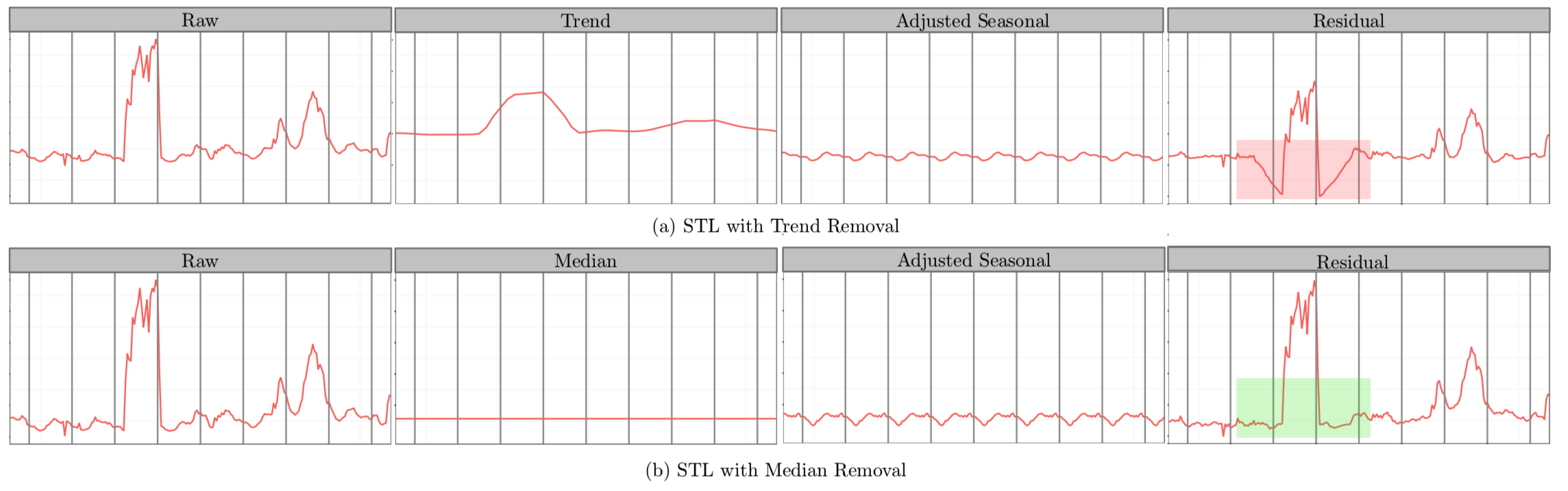# 1. 基于统计的异常检测

## Grubbs' Test

Grubbs' Test为一种假设检验的方法，常被用来检验服从正太分布的单变量数据集（univariate data set）$Y$ 中的单个异常值。若有异常值，则其必为数据集中的最大值或最小值。原假设与备择假设如下：

$H_0$: 数据集中没有异常值
$H_1$: 数据集中有一个异常值

Grubbs' Test检验假设的所用到的检验统计量（test statistic）为

$G = \frac{\max |Y_i - \overline{Y}|}{s}$

$G > \frac{(N-1)}{\sqrt{N}}\sqrt{\frac{ (t_{\alpha/(2N), N-2})^2}{N-2 + (t_{\alpha/(2N), N-2})^2}}$

## ESD

• 计算与均值偏离最远的残差，注意计算均值时的数据序列应是删除上一轮最大残差样本数据后；

\begin{equation}
R_j = \frac{\max_i |Y_i - \overline{Y'}|}{s}, \quad 1 \leq j \leq k
\label{eq:esd_test}
\end{equation}

• 计算临界值（critical value）；

$\lambda_j = \frac{(n-j) * t_{p,n-j-1}}{\sqrt{(n-j-1+t_{p,n-j-1}^2)(n-j+1)}}, \quad 1 \leq j \leq k$

• 检验原假设，比较检验统计量与临界值；若$R_i > \lambda_j$，则原假设$H_0$不成立，该样本点为异常点；

• 重复以上步骤$k$次至算法结束。

# 2. 时间序列的异常检测

## S-ESD

STL将时间序列数据分解为趋势分量、周期分量和余项分量。想当然的解法——将ESD运用于STL分解后的余项分量中，即可得到时间序列上的异常点。但是，我们会发现在余项分量中存在着部分假异常点（spurious anomalies）。如下图所示：$R_X = X - S_X- \tilde{X}$

## S-H-ESD

$MAD = median(|X_i - median(X)|)$

S-H-ESD的Python实现有pyculiarity
，时间序列异常检测数据集有Yahoo公开的A Labeled Anomaly Detection Dataset

# 3. 参考资料

 Hochenbaum, Jordan, Owen S. Vallis, and Arun Kejariwal. "Automatic Anomaly Detection in the Cloud Via Statistical Learning." arXiv preprint arXiv:1704.07706 (2017).

posted @ 2018-06-20 10:48  Treant  阅读(11902)  评论(2编辑  收藏